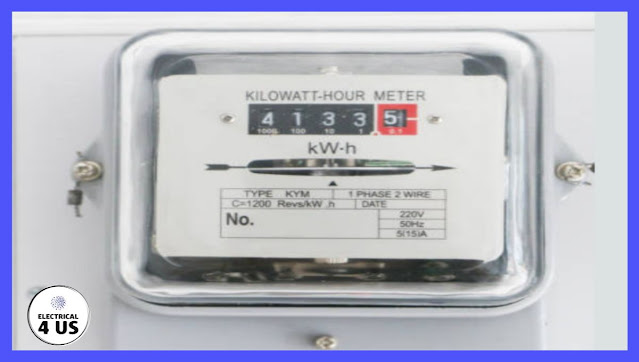## Posts

Showing posts from February, 2022

### How does an electric metre work?How does an electric metre work? Electric Meter It is designed to measure kWh, and to do so it measures Voltage (Across Incoming Line) Current (probably only in one leg, as the other would be symmetric with it) Phase (offset between voltage sine wave and current sine wave) Cable suitable for measuring current has to physically pass through a part of the equipment - usually a current transformer is placed around the current-carrying conductor. The most convenient designs have four large connection terminals - two in and two out (some more in 3-phase meters, of course) to make wiring operations easier. One can think of the meter as a black box in series with the supply, if that helps. Single phase Meter The instrument starts by continuously measuring the instantaneous power, using the calculations: Power = Voltage × Current × Cosine (θ) where is the phase offset measured between the two waves. To convert this to kWh (which we pay for) the instantaneous power is integrated over time